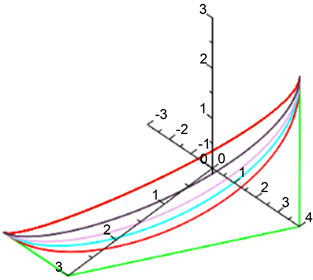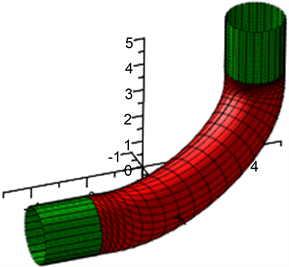﻿ 三次有理B样条曲线及其应用

三次有理B样条曲线及其应用Cubic Rational B-Spline Curve and Its Application

Abstract: In this paper, we construct a section of cubic rational B-spline curve which is tangent to the start and end points of the control polygon. Tubes whose axes are in non-coplaner are smoothly blended by tube take cubic rational B-spline curve as its axis. Compared with the three-segment smooth  blending tubes with three-segment continuous cubic uniform B-spline curves as the axis between tubes whose axes are non-coplana, and the two-segment smooth blending tubes with quadratic uniform B-spline curves as the axis between tubes whose axes are non-coplana, the proposed method has the advantages of simple structure and easy application.

1. 引言

2. 过指定顶点的三次有理B样条曲线及其性质

$r\left(t\right)={\sum }_{i=0}^{3}{N}_{i,3}\left(t\right){V}_{i+1}$

$r\left(t\right)=\frac{\underset{j=0}{\overset{3}{\sum }}{N}_{j,3}\left(t\right){\omega }_{j}{V}_{i+j}}{\underset{j=0}{\overset{3}{\sum }}{N}_{j,3}\left(t\right){\omega }_{j}}$

$r\left(t\right)=\frac{\underset{j=0}{\overset{3}{\sum }}{N}_{j,3}\left(t,{\omega }_{j}\right){V}_{i+j}}{\underset{j=0}{\overset{3}{\sum }}{N}_{j,3}\left(t,{\omega }_{j}\right)}$

${P}_{0},{P}_{1},{P}_{2},{P}_{3}$ 是给定的三次均匀B样条曲线的控制多边形的顶点，构造一段实际控制顶点为 ${V}_{0},{V}_{1},{V}_{2},{V}_{3}$ 的三次均匀有理B样条曲线，使得端点是 ${P}_{0}$${P}_{3}$，且 ${P}_{0}$${P}_{3}$ 处的切线分别为 ${P}_{1}-{P}_{0}$${P}_{3}-{P}_{2}$，则

$\left\{\begin{array}{l}r\left(0\right)={P}_{0},\\ r\left(1\right)={P}_{3},\\ {r}^{\prime }\left(0\right)={P}_{1}-{P}_{0},\\ {r}^{\prime }\left(1\right)={P}_{3}-{P}_{2}.\end{array}$

$\left\{\begin{array}{l}{V}_{0}=\frac{\left(25{w}_{0}+16{w}_{1}-17{P}_{0}{w}_{2}\right){P}_{0}+\left(-7{w}_{0}-28{w}_{1}-7{w}_{2}\right){P}_{1}+\left(2{w}_{1}+8{w}_{2}+2{w}_{3}\right){P}_{2}+\left(10{w}_{1}+16{w}_{2}-2{w}_{3}\right){P}_{3}}{18{w}_{0}},\\ {V}_{1}=-\frac{\left(2{w}_{0}-16{w}_{1}-10{w}_{2}\right){P}_{0}+\left(-2{w}_{0}-8{w}_{1}-2{w}_{2}\right){P}_{1}+\left({w}_{1}+4{w}_{2}+{w}_{2}\right){P}_{2}+\left(5{w}_{3}+5{w}_{1}+8{w}_{2}-{w}_{3}\right){P}_{3}}{18{w}_{1}},\\ {V}_{2}=\frac{\left({w}_{0}-8{w}_{1}-5{w}_{2}\right){P}_{0}+\left(-{w}_{0}-4{w}_{1}-{w}_{2}\right){P}_{1}+\left(2{w}_{1}+8{w}_{2}+2{w}_{3}\right){P}_{2}+\left(10{w}_{1}+16{P}_{3}{w}_{2}-2{w}_{3}\right){P}_{3}}{18{w}_{2}},\\ {V}_{3}=-\frac{\left(2{w}_{0}-16{w}_{1}-10{w}_{2}\right){P}_{0}+\left(-2{w}_{0}-8{w}_{1}-2{w}_{2}\right){P}_{1}+\left(7{w}_{1}+28{w}_{2}+7{w}_{3}\right){P}_{2}+\left(17{w}_{1}-16{w}_{2}-25{w}_{3}\right){P}_{3}}{18{w}_{3}}.\end{array}$ (1)

${P}_{0},{P}_{1},{P}_{2},{P}_{3}$ 的坐标 $\left({P}_{1,j},{P}_{2,j},{P}_{3,j}\right)\left(j=0,1,2,3\right)$，代入，可得所求三次均匀有理B样条曲线的实际控制顶点 ${V}_{0}\left({x}_{0},{y}_{0},{z}_{0}\right)$${V}_{1}\left({x}_{1},{y}_{1},{z}_{1}\right)$${V}_{2}\left({x}_{2},{y}_{2},{z}_{2}\right)$${V}_{3}\left({x}_{3},{y}_{3},{z}_{3}\right)$，则所求三次均匀有理B样条曲线的参数表达式为

$\left\{\begin{array}{l}x\left(t,w\right)=\frac{\underset{j=0}{\overset{3}{\sum }}{N}_{j,3}\left(t,{w}_{j}\right){x}_{j}}{\underset{j=0}{\overset{3}{\sum }}{N}_{j,3}\left(t,{w}_{j}\right)},\\ y\left(t,w\right)=\frac{\underset{j=0}{\overset{3}{\sum }}{N}_{j,3}\left(t,{w}_{j}\right){y}_{j}}{\underset{j=0}{\overset{3}{\sum }}{N}_{j,3}\left(t,{w}_{j}\right)},\\ z\left(t,w\right)=\frac{\underset{j=0}{\overset{3}{\sum }}{N}_{j,3}\left(t,{w}_{j}\right){z}_{j}}{\underset{j=0}{\overset{3}{\sum }}{N}_{j,3}\left(t,{w}_{j}\right)}.\end{array}t\in \left[0,1\right]$

$\left\{\begin{array}{l}{x}_{0}=\frac{54{\omega }_{0}-36{\omega }_{1}-72{\omega }_{2}}{18{\omega }_{0}},\\ {y}_{0}=\frac{-75{\omega }_{0}+147{\omega }_{2}}{18{\omega }_{0}},\\ {z}_{0}=\frac{30{\omega }_{1}+48{\omega }_{2}-6{\omega }_{3}}{18{\omega }_{0}}.\end{array}$ $\left\{\begin{array}{l}{x}_{1}=\frac{4{\omega }_{1}+2{\omega }_{2}}{{\omega }_{1}},\\ {y}_{1}=-\frac{-6{\omega }_{0}+72{\omega }_{1}+78{\omega }_{2}}{18{\omega }_{1}},\\ {z}_{1}=-\frac{15{\omega }_{1}+24{\omega }_{2}-3{\omega }_{3}}{18{\omega }_{1}}.\end{array}$

$\left\{\begin{array}{l}{x}_{2}=-\frac{2{\omega }_{1}+{\omega }_{2}}{{\omega }_{2}},\\ {y}_{2}=\frac{-3{\omega }_{0}+72{\omega }_{1}+111{\omega }_{2}}{18{\omega }_{2}},\\ {z}_{2}=\frac{30{\omega }_{1}+48{\omega }_{2}-6{\omega }_{3}}{18{\omega }_{2}}.\end{array}$ $\left\{\begin{array}{l}{x}_{3}=\frac{4{\omega }_{1}+2{\omega }_{2}}{{\omega }_{3}},\\ {y}_{3}=\frac{6{\omega }_{0}-144{\omega }_{1}-78{\omega }_{2}+{72}_{3}}{18{\omega }_{3}},\\ {z}_{0}=\frac{-51{\omega }_{1}+48{\omega }_{2}+75{\omega }_{3}}{18{\omega }_{3}}.\end{array}$

(1) 当 $|{\omega }_{0}|=|{\omega }_{1}|=|{\omega }_{2}|=|{\omega }_{3}|\ge 1$${\omega }_{0},{\omega }_{1},{\omega }_{2},{\omega }_{3}<0$ 时，是一条过 ${P}_{0}$${P}_{3}$，且 ${P}_{0}$${P}_{3}$ 处的切线分别为 ${P}_{1}-{P}_{0}$${P}_{3}-{P}_{2}$ 的实际控制顶点为 ${V}_{0}\left({x}_{0},{y}_{0},{z}_{0}\right)$${V}_{1}\left({x}_{1},{y}_{1},{z}_{1}\right)$${V}_{2}\left({x}_{2},{y}_{2},{z}_{2}\right)$${V}_{3}\left({x}_{3},{y}_{3},{z}_{3}\right)$ 的三次均匀B样条曲线。

(2) 当 ${\omega }_{0}={\omega }_{3}=1,0<{\omega }_{1},{\omega }_{2}<1$ 时，是一条过 ${P}_{0}$${P}_{3}$，且 ${P}_{0}$${P}_{3}$ 处的切线分别为 ${P}_{1}-{P}_{0}$${P}_{3}-{P}_{2}$ 的实际控制顶点为 ${V}_{0}\left({x}_{0},{y}_{0},{z}_{0}\right)$${V}_{1}\left({x}_{1},{y}_{1},{z}_{1}\right)$${V}_{2}\left({x}_{2},{y}_{2},{z}_{2}\right)$${V}_{3}\left({x}_{3},{y}_{3},{z}_{3}\right)$ 的三次有理B样条曲线， ${\omega }_{1},{\omega }_{2}$ 越小，曲线越靠近控制多边形的边 ${P}_{1}{P}_{2}$Figure 1. The adjustment function of rational B-spline curve with different weight factor ${\omega }_{0},{\omega }_{1},{\omega }_{2},{\omega }_{3}$

3. 过指定顶点的三次有理B样条曲线及其在轴线异面管道拼接中的应用

${\Phi }_{1}\left(t,\phi \right):\left\{\begin{array}{l}x\left(t,\phi \right)=3+a{e}_{11}\cdot \mathrm{sin}\phi +a{e}_{21}\cdot \mathrm{cos}\phi ,\\ y\left(t,\phi \right)=-3+t+a{e}_{12}\cdot \mathrm{sin}\phi +a{e}_{22}\cdot \mathrm{cos}\phi ,\\ z\left(t,\phi \right)=0+a{e}_{13}\cdot \mathrm{sin}\phi +a{e}_{23}\cdot \mathrm{cos}\phi .\end{array}t\in \left[0,1\right],\phi \in \left[0,2\pi \right]$

${\Phi }_{2}\left(t,\phi \right):\left\{\begin{array}{l}x\left(t,\phi \right)=0+a{{e}^{\prime }}_{11}\cdot \mathrm{sin}\phi +a{{e}^{\prime }}_{21}\cdot \mathrm{cos}\phi ,\\ y\left(t,\phi \right)=4+a{{e}^{\prime }}_{12}\cdot \mathrm{sin}\phi +a{{e}^{\prime }}_{22}\cdot \mathrm{cos}\phi ,\\ z\left(t,\phi \right)=3+t+a{{e}^{\prime }}_{13}\cdot \mathrm{sin}\phi +a{{e}^{\prime }}_{23}\cdot \mathrm{cos}\phi .\end{array}t\in \left[0,1\right],\phi \in \left[0,2\pi \right]$

$\Phi \left(t,\phi \right):\left\{\begin{array}{l}x\left(t,\phi \right)=x\left(t\right)+a{B}_{1}\left(t\right)\mathrm{sin}\phi +a{N}_{1}\left(t\right)\mathrm{cos}\phi ,\\ y\left(t,\phi \right)=y\left(t\right)+a{B}_{2}\left(t\right)\mathrm{sin}\phi +a{N}_{2}\left(t\right)\mathrm{cos}\phi ,\\ z\left(t,\phi \right)=z\left(t\right)+a{B}_{3}\left(t\right)\mathrm{sin}\phi +a{N}_{3}\left(t\right)\mathrm{cos}\phi .\end{array}t\in \left[0,1\right],\phi \in \left[0,2\pi \right]$

$\gamma \left(t\right):\left\{\begin{array}{l}x\left(t\right)=-\frac{3\left(37{t}^{3}-129{t}^{2}+147t-55\right)}{147{t}^{2}-147t+55},\\ y\left(t\right)=\frac{424{t}^{3}-645{t}^{2}+666t-165}{147{t}^{2}-147t+55},\\ z\left(t\right)=\frac{276{t}^{3}-111{t}^{2}}{147{t}^{2}-147t+55}.\end{array}t\in \left[0,1\right]$Figure 2. When, ${\omega }_{0}={\omega }_{3}=1,{\omega }_{1}={\omega }_{2}=\frac{1}{50}$, the effect drawing of circular tube is the same radiuses tube with cubic rational B-spline curve as the axis

${\Phi }_{1}\left(t,\phi \right):\left\{\begin{array}{l}x\left(t,\phi \right)=3+a{e}_{11}\cdot \mathrm{sin}\phi +a{e}_{21}\cdot \mathrm{cos}\phi ,\\ y\left(t,\phi \right)=-3+t+a{e}_{12}\cdot \mathrm{sin}\phi +a{e}_{22}\cdot \mathrm{cos}\phi ,\\ z\left(t,\phi \right)=0+a{e}_{13}\cdot \mathrm{sin}\phi +a{e}_{23}\cdot \mathrm{cos}\phi .\end{array}t\in \left[0,1\right],\phi \in \left[0,2\pi \right]$

${\Phi }_{2}\left(t,\phi \right):\left\{\begin{array}{l}x\left(t,\phi \right)=0+b{{e}^{\prime }}_{11}\cdot \mathrm{sin}\phi +b{{e}^{\prime }}_{21}\cdot \mathrm{cos}\phi ,\\ y\left(t,\phi \right)=4+b{{e}^{\prime }}_{12}\cdot \mathrm{sin}\phi +b{{e}^{\prime }}_{22}\cdot \mathrm{cos}\phi ,\\ z\left(t,\phi \right)=3+t+b{{e}^{\prime }}_{13}\cdot \mathrm{sin}\phi +b{{e}^{\prime }}_{23}\cdot \mathrm{cos}\phi .\end{array}t\in \left[0,1\right],\phi \in \left[0,2\pi \right]$

$\Phi \left(t,\phi \right)=\left\{\begin{array}{l}x\left(t\right)+d\left(t\right){N}_{1}\left(t\right)\mathrm{cos}\phi +d\left(t\right){B}_{1}\left(t\right)\mathrm{sin}\phi ,\\ y\left(t\right)+d\left(t\right){N}_{2}\left(t\right)\mathrm{cos}\phi +d\left(t\right){B}_{2}\left(t\right)\mathrm{sin}\phi ,\\ z\left(t\right)+d\left(t\right){N}_{3}\left(t\right)\mathrm{cos}\phi +d\left(t\right){B}_{3}\left(t\right)\mathrm{sin}\phi .\end{array}t\in \left[0,1\right],\phi \in \left[0,2\pi \right].$

${\gamma }^{\prime }\left(t\right)=\left\{\begin{array}{l}{x}^{\prime }\left(t\right)=-\frac{2\left(49{t}^{3}-165{t}^{2}+183t-67\right)}{147{t}^{2}-147t+55},\\ {y}^{\prime }\left(t\right)=\frac{360{t}^{3}-681{t}^{2}+606t-165}{147{t}^{2}-147t+55},\\ {z}^{\prime }\left(t\right)=\frac{276{t}^{3}-111{t}^{2}}{147{t}^{2}-147t+55}.\end{array}t\in \left[0,1\right]$Figure 3. When, ${\omega }_{0}={\omega }_{3}=1,{\omega }_{1}={\omega }_{2}=\frac{1}{50}$, the effect drawing of circular tube is the different radiuses tube with cubic rational B-spline curve as the axis

4. 结束语

 Wang, H. and Bai, G.Z. (2013) Employing Generalized Cylindrical Helicoid Tube to Smoothly Blending Tubes Whose Axes Are Non-Coplanar. Applied Mechanics and Materials, 380-384, 1750-1754.
https://doi.org/10.4028/www.scientific.net/AMM.380-384.1750

 Bai, G.-Z., Wang, H., et al. (2014) Smoothly Blending of Two Elliptic Cylinders Whose Axes Are Non-Coplanar. Aplied Mechanics and Materials, 309-312, 644-650.
https://doi.org/10.4028/www.scientific.net/AMM.644-650.309

 Bai, G.-Z., Liu, S.-Y., Wang, H., W, Z. and Zhao, J.-Y. (2014) A Novel Method for Smooth Blending Cylindrical Surfaces Whose Axes Are Non-Coplanar Based on Smooth Blending Axes. Applied Mechanics and Materials, 687-691, 1470-1473.
https://doi.org/10.4028/www.scientific.net/AMM.687-691.1470

 Bai, G.Z., Wu, Z. and Lin, X. (2017) Intersecting Line of Conical Surface and Smoothly Blending of Two Tubes Whose Axes Are Non-Coplanar. Journal of Applied Mathematics and Physics, 5, 1887-1991.
https://doi.org/10.4236/jamp.2017.59158

 Bai, G.Z., Wang, H. and Yin, Z.J. (2014) Employing Generalized Bezier Tube to Smoothly Blending Tubes Whose Axes Are Non-Coplanar. Applied Mechanics and Materials, 513-517, 2301-2306.
https://doi.org/10.4028/www.scientific.net/AMM.513-517.2301

 Bai, G.Z. and Wang, F. (2019) Application of Quasi-Cubic Bezier Curves in the Blending of Tubes with Different Radiuses. Materials Science and Engineering, Vol. 612, 1-7.
https://doi.org/10.1088/1757-899X/612/3/032171

 白根柱. 基于轴线光滑拼接的轴线异面管道拼接技术[J]. 应用数学进展, 2019, 8(7): 1267-1271.

Top